### Home > CCA > Chapter 9 > Lesson 9.1.1 > Problem9-6

9-6.
1. Use your generalized process of completing the square to rewrite and solve each quadratic equation below. Homework Help ✎

1. w2 + 28w + 52 = 0

2. x2 + 5x + 4 = 0

3. k2 − 16k − 17 = 0

4. z2 − 1000z + 60775 = 0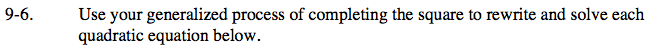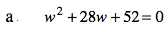Draw tiles to make the expression into a square.

How many tiles do you need to complete the square?

Add that number to both sides of the equation and simplify.

(w + 14)2 = 144

Solve for w by starting with eliminating the exponent.

w = −2 or w = −26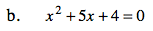See part (a).

(x + 2.5)2 = 2.25
x = −1 or x = −4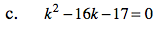See part (a).

(k − 8)2 = 81
k = 17 or k = −1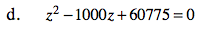See part (a).